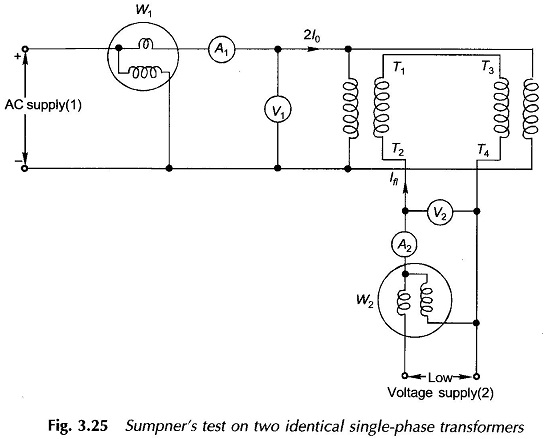# Sumpner Test

## Sumpner Test (Back-to-Back):

While OC and SC tests on a transformer yield its equivalent circuit parameters, these cannot be used for the ‘heat run’ test wherein the purpose is to determine the steady temperature rise if the transformer was fully loaded continuously; this is so because under each of these tests the power loss to which the transformer is subjected is either the core-loss or copper-loss but not both. The way out of this impasse without conducting an actual loading test is the Sumpner Test which can only be conducted simultaneously on two identical transformers.

In conducting the Sumpner Test the primaries of the two transformers are connected in parallel across the rated voltage supply (V1), while the two secondaries are connected in phase opposition as shown in Fig. 3.25.

For the secondaries to be in phase opposition, the voltage across T2T4 must be zero otherwise it will be double the rated secondary voltage in which case the polarity of one of the secondaries must be reversed. Current at low voltage (V2) is injected into the secondary circuit at T2T4 .As per the superposition theorem, if V2 source is assumed shorted, the two transformers appear in open-circuit to source V1 as their secondaries are in phase opposition and therefore no current can flow in them.

The current drawn from source V1 is thus 2I0 (twice the no-load current of each transformer) and power is 2P0 (= 2Pi, twice the core-loss of each transformer). When V1 is regarded as shorted, the transformers are series-connected across V2 and are short-circuited on the side of primaries. Therefore, the impedance seen at V2 is 2Z and when V2 is adjusted to circulate full-load current (Ifl), the power fed in is 2Pc (twice the full-load copper-loss of each transformer).

Thus in the Sumpner Test while the transformers are not supplying any load, full iron-loss occurs in their cores and full copper-loss occurs in their windings; net power input to the transformers being (2P0 + 2Pc). The heat run test could, therefore, be conducted on the two transformers, while only losses are supplied.

In Fig. 3.25 the auxiliary voltage source is included in the circuit of secondaries; the test could also be conducted by including the auxiliary source in the circuit of primaries.

Updated: August 26, 2019 — 10:52 pm

`Please enable JavaScript in your browser to complete this form.Name *FirstLastEmail *Paragraph TextMessage`
` `